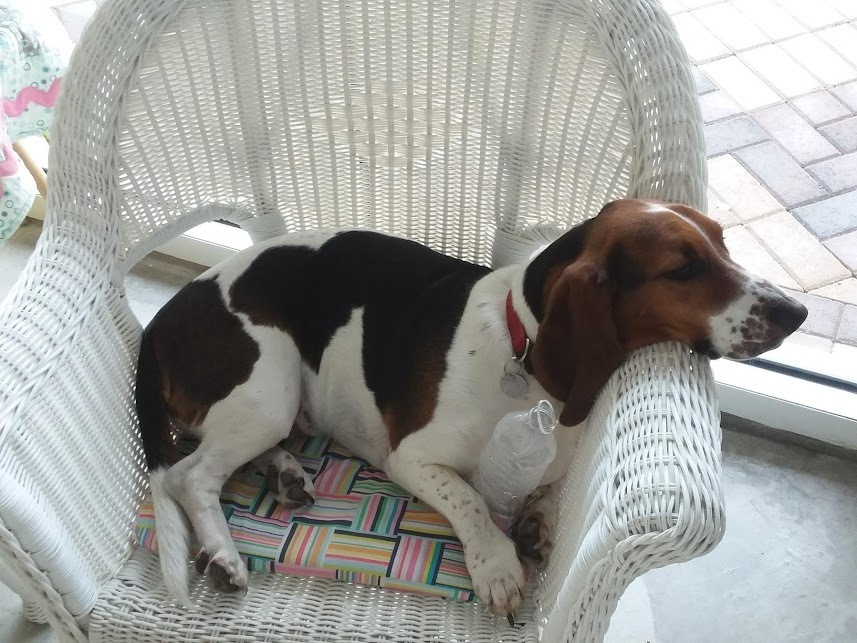# Stanley's Birthday is this weekend!

He's wondering if anyone will come and sing to him!
Stop in this Friday or Saturday to wish Stanley a Happy 7th Birthday and give our Prize Wheel a spin!

 table div table+table+table+table div table{width:100%;padding:0}table div table+table+table+table div table img{width:96.23%;padding:0;float:none}table div table+table+table+table div table td{width:100%;padding:0 1.88% 18px}/* styles */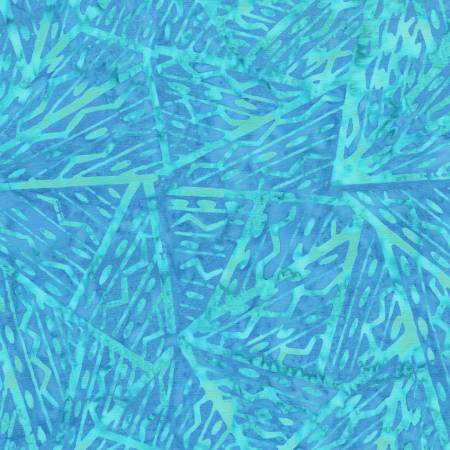# Colorful Anthology Batiks!

If you love bright, clear Batiks, then you will love the 30 new Batiks from Anthology!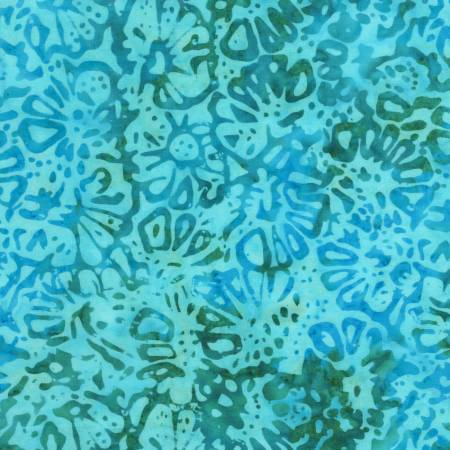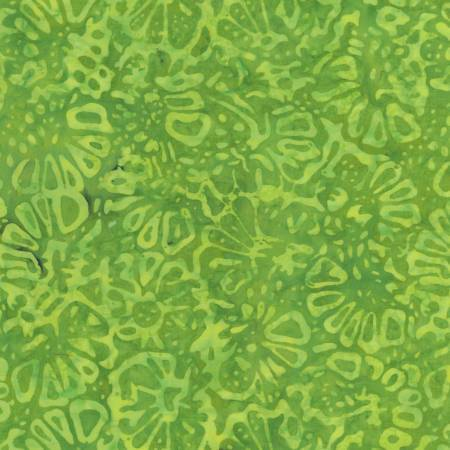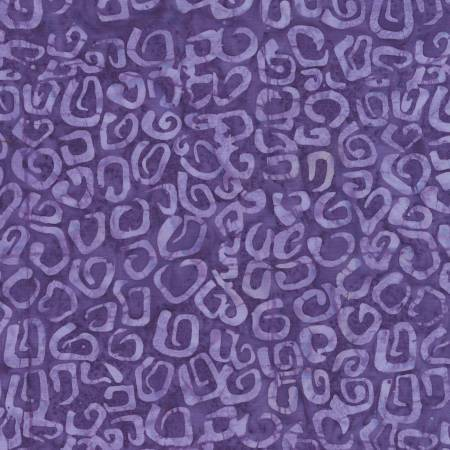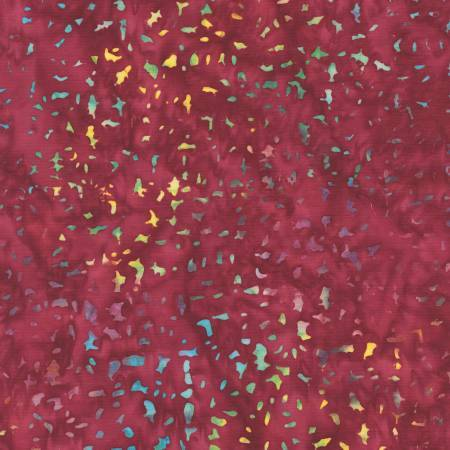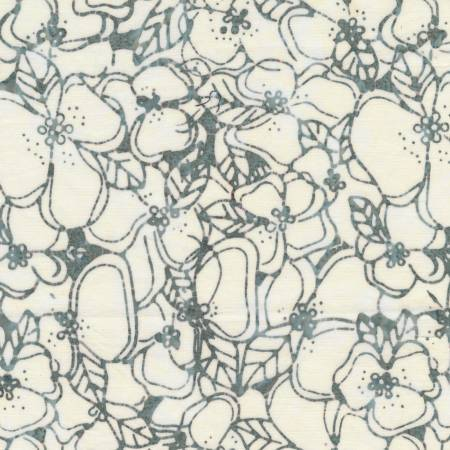table div table+table+table+table+table+table+table+table+table div table{width:100%;padding:0}table div table+table+table+table+table+table+table+table+table div table img{width:96.23%;padding:0;float:none}table div table+table+table+table+table+table+table+table+table div table td{width:100%;padding:0 1.88% 18px}/* styles */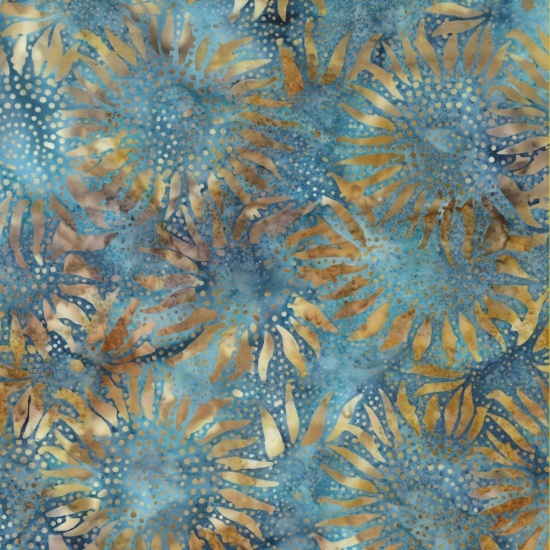# A Deep, Rich Color Palette from Hoffman!

Deep Blues, luscious greens and even rich browns make up this sophisticated palette of Hoffman Batiks!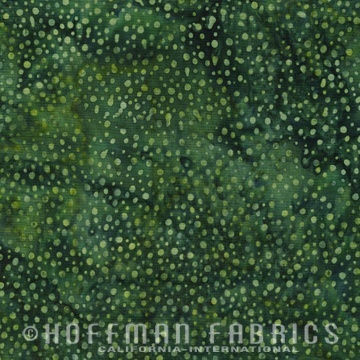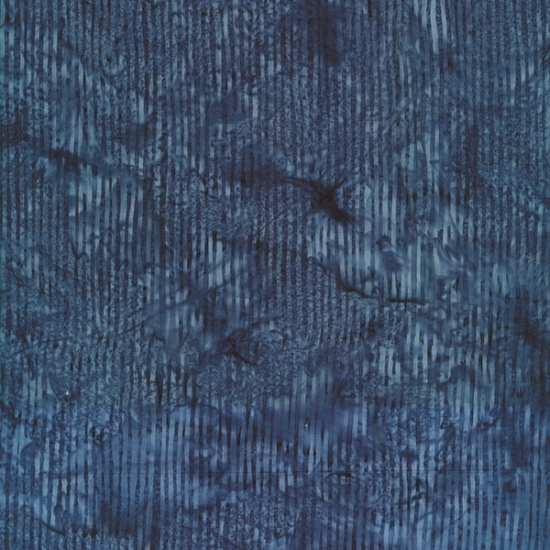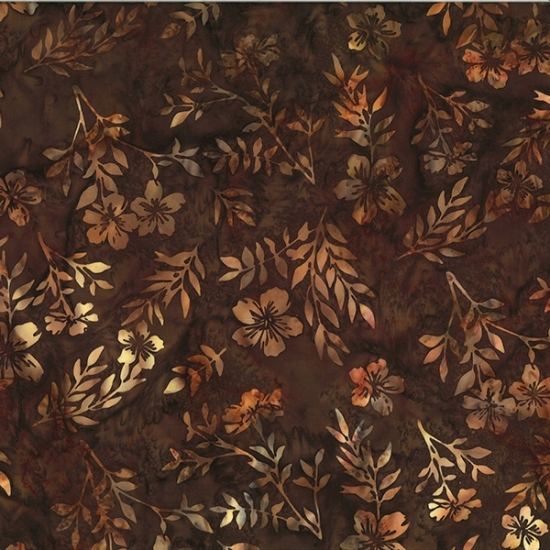table div table+table+table+table+table+table+table+table+table+table+table+table+table div table{width:100%;padding:0}table div table+table+table+table+table+table+table+table+table+table+table+table+table div table img{width:96.23%;padding:0;float:none}table div table+table+table+table+table+table+table+table+table+table+table+table+table div table td{width:100%;padding:0 1.88% 18px}/* styles */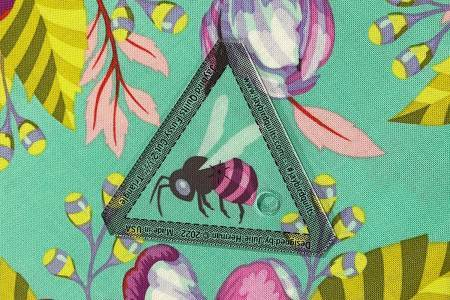# New Notions and Patterns!

Fussy Cut Shapes from Jaybird Quilts are here! These 6 acrylic templates work with the Hex N More and Sidekick ruler shapes for all your fussy cutting fun!
Super cute aqua magnetic pincushion that comes with red pins!
New patterns to use those fat quarters you've been looking at!
Be sure to check out all the new goodies!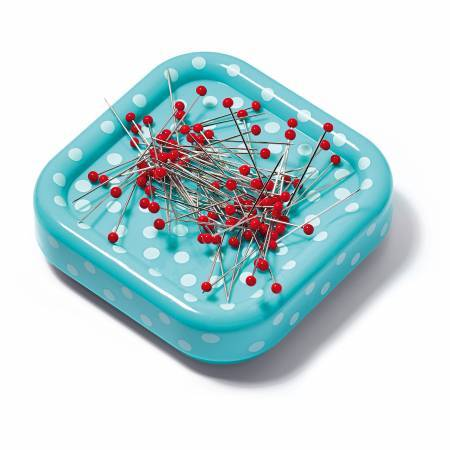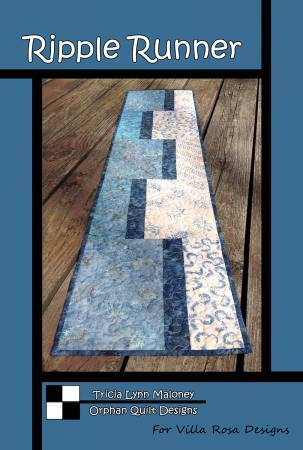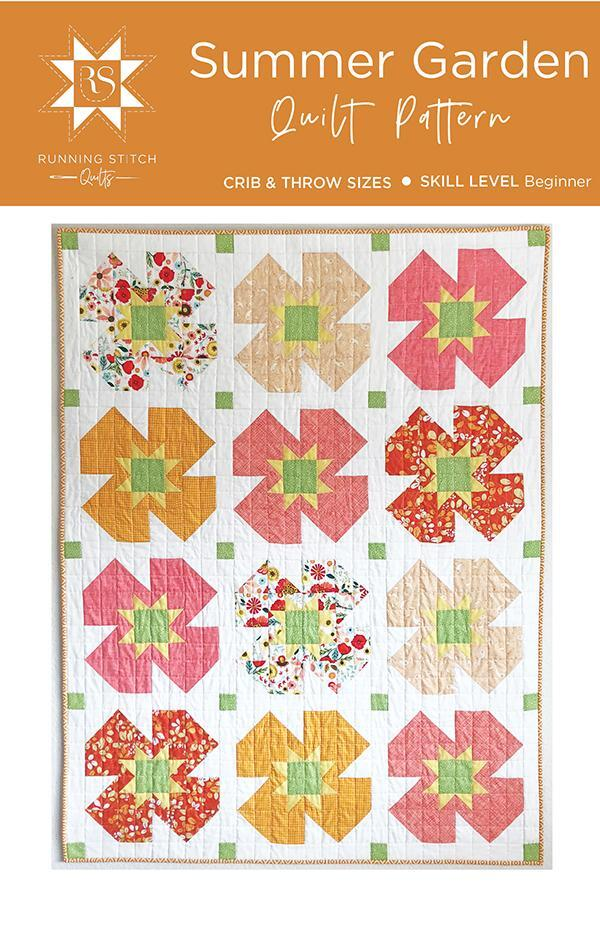table div table+table+table+table+table+table+table+table+table+table+table+table+table+table+table+table+table div table{width:100%;padding:0}table div table+table+table+table+table+table+table+table+table+table+table+table+table+table+table+table+table div table img{width:96.23%;padding:0;float:none}table div table+table+table+table+table+table+table+table+table+table+table+table+table+table+table+table+table div table td{width:100%;padding:0 1.88% 18px}/* styles */## By Annie Trunk Show!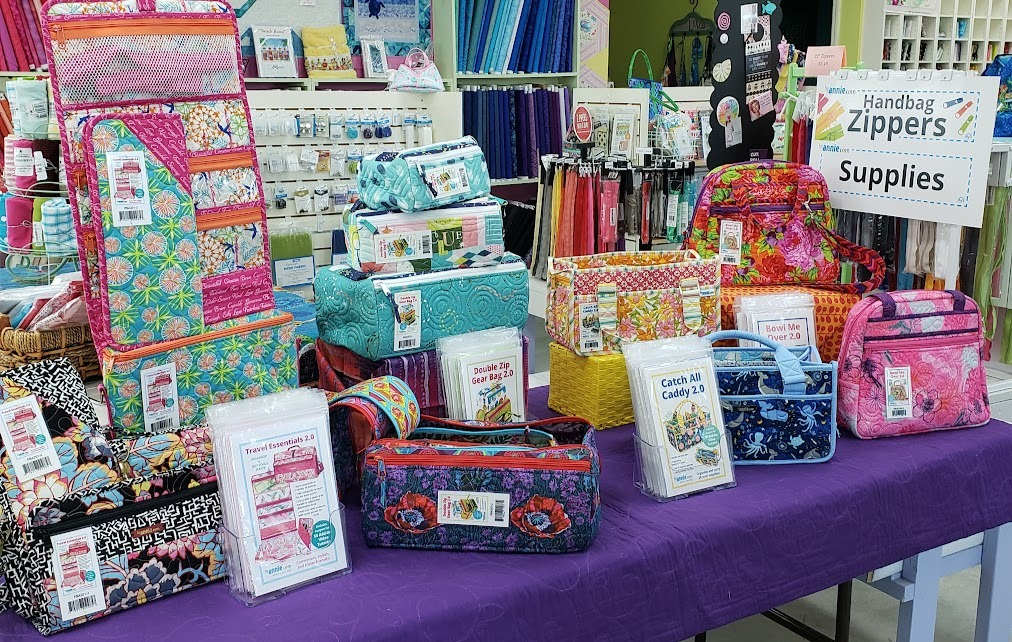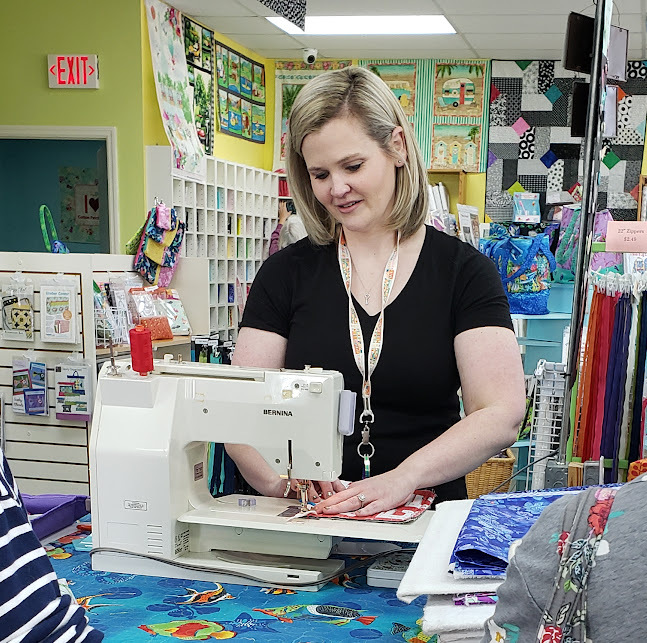We have borrowed beautifully constructed bag samples from By Annie herself for an awesome trunk show which will be here through the end of the month.

These are the 4 bags that Madison will be teaching a virtual bag class series on beginning in February.

 table div table+table+table+table+table+table+table+table+table+table+table+table+table+table+table+table+table+table+table+table+table+table div table{width:100%;padding:0}table div table+table+table+table+table+table+table+table+table+table+table+table+table+table+table+table+table+table+table+table+table+table div table img{width:96.23%;padding:0;float:none}table div table+table+table+table+table+table+table+table+table+table+table+table+table+table+table+table+table+table+table+table+table+table div table td{width:100%;padding:0 1.88% 18px}/* styles */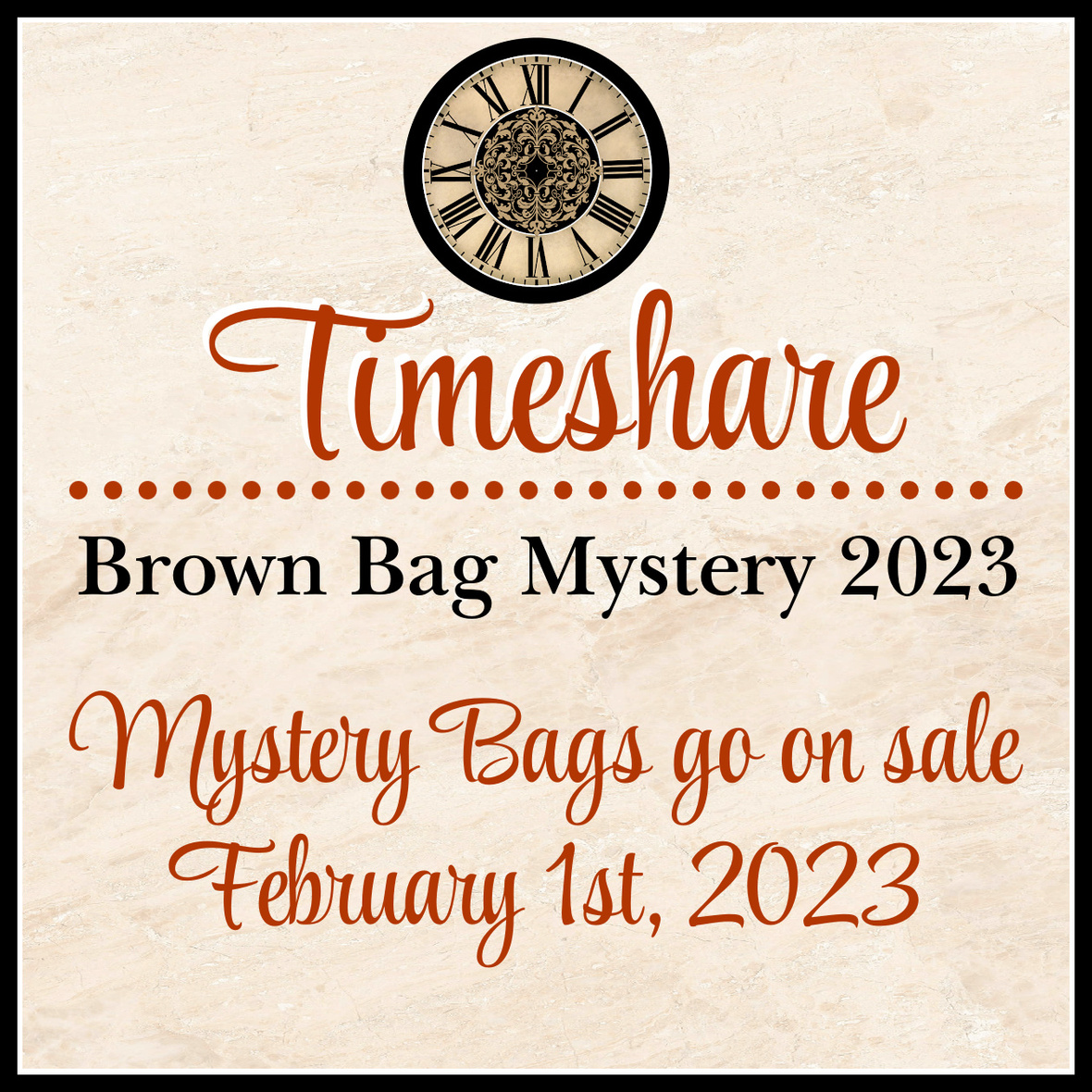# The Brown Bag Mystery!

Cotton Patch is a Sewposium Shop and we will be participating in the Brown Bag Mystery in 2023!

Bags go on sale February 1 and the first clue is March 18.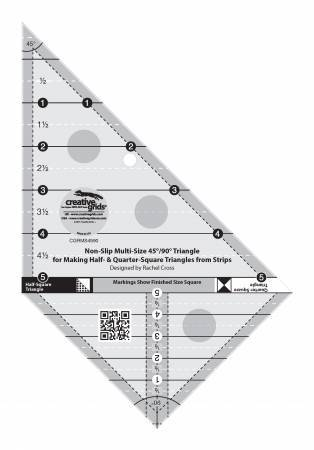The ruler Karen is recommending (not required) for the Brown Bag Mystery is the Creative Grids Multi Size Triangle 45 and 90 Degrees Ruler and we have them in stock!

 table div table+table+table+table+table+table+table+table+table+table+table+table+table+table+table+table+table+table+table+table+table+table+table+table+table+table div table{width:100%;padding:0}table div table+table+table+table+table+table+table+table+table+table+table+table+table+table+table+table+table+table+table+table+table+table+table+table+table+table div table img{width:96.23%;padding:0;float:none}table div table+table+table+table+table+table+table+table+table+table+table+table+table+table+table+table+table+table+table+table+table+table+table+table+table+table div table td{width:100%;padding:0 1.88% 18px}/* styles */# NO Sunday Soiree on January 22 (Laura will be at Sewposium)# Sunday Soiree!

## FB Live is the place to be Sunday at 7pm!

You will be the 1st to see new fabrics, notions, kits and other great things that you need to know about!
We put together fun kits that often sell out that very night - you don't want to miss out!

There will be prizes, live demos and special pricing!

You will be able to purchase during the Live Broadcast and we can ship - sort of like Stuff Your Stocking but all in one night.
If you miss the FB Live - not to worry!
You can view the replay on our FB Page AND you can still purchase at the special prices until 12pm Monday!

Join me for a fun evening!

 table div table+table+table+table+table+table+table+table+table+table+table+table+table+table+table+table+table+table+table+table+table+table+table+table+table+table+table+table+table+table+table div table{width:100%;padding:0}table div table+table+table+table+table+table+table+table+table+table+table+table+table+table+table+table+table+table+table+table+table+table+table+table+table+table+table+table+table+table+table div table img{width:96.23%;padding:0;float:none}table div table+table+table+table+table+table+table+table+table+table+table+table+table+table+table+table+table+table+table+table+table+table+table+table+table+table+table+table+table+table+table div table td{width:100%;padding:0 1.88% 18px}/* styles */# There's a lot going on at Cotton Patch that you won't want to miss!

January 14, By Annie Trunk Show with Madison in town!
January 21, Special Saturday Night FB Live in place of 1/22
January 22, Stanley's Birthday
February 1 at 12:01am, Brown Bag Mystery goes on sale!
March 1, All State Florida Shop Hop Begins
March 18, first clue for Brown Bag Mystery
April 1, Sewing Sewcial Blocks go on sale

 table div table+table+table+table+table+table+table+table+table+table+table+table+table+table+table+table+table+table+table+table+table+table+table+table+table+table+table+table+table+table+table+table+table div table{width:100%;padding:0}table div table+table+table+table+table+table+table+table+table+table+table+table+table+table+table+table+table+table+table+table+table+table+table+table+table+table+table+table+table+table+table+table+table div table img{width:96.23%;padding:0;float:none}table div table+table+table+table+table+table+table+table+table+table+table+table+table+table+table+table+table+table+table+table+table+table+table+table+table+table+table+table+table+table+table+table+table div table td{width:100%;padding:0 1.88% 18px}/* styles */We are open on Tuesday, Wednesday, Thursday, Friday and Saturday from 10am to 4pm for instore shopping!

All of our staff are vaccinated.
Masks are not required at this time. We look forward to your visit!

 table div table+table+table+table+table+table+table+table+table+table+table+table+table+table+table+table+table+table+table+table+table+table+table+table+table+table+table+table+table+table+table+table+table+table+table div table{width:100%;padding:0}table div table+table+table+table+table+table+table+table+table+table+table+table+table+table+table+table+table+table+table+table+table+table+table+table+table+table+table+table+table+table+table+table+table+table+table div table img{width:96.23%;padding:0;float:none}table div table+table+table+table+table+table+table+table+table+table+table+table+table+table+table+table+table+table+table+table+table+table+table+table+table+table+table+table+table+table+table+table+table+table+table div table td{width:100%;padding:0 1.88% 18px}/* styles *//* styles */ It's butt to butt bassets!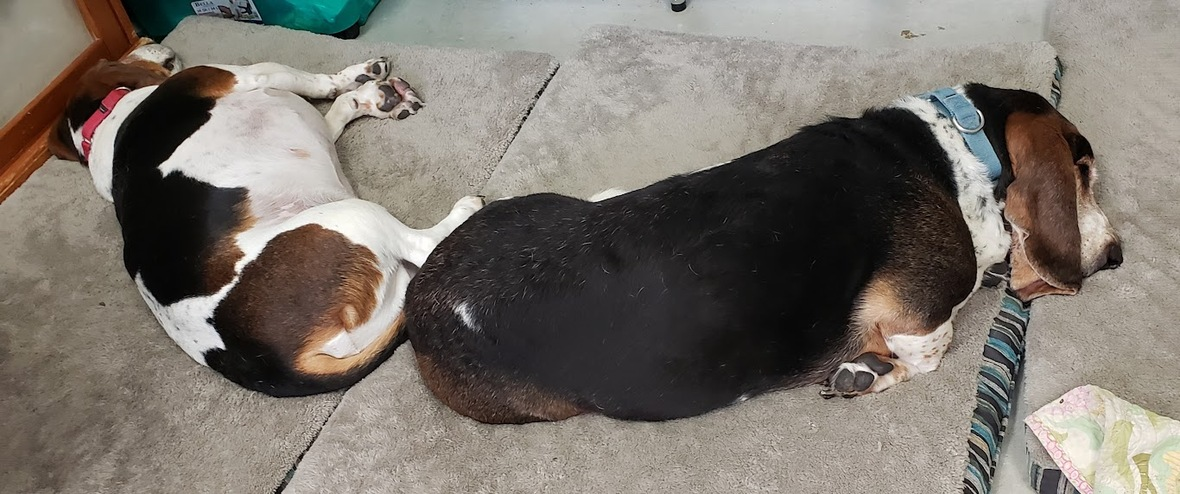Like   Pin   +1